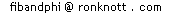# Using Powers of Phi to represent Integers (Base Phi)

If you have already looked at the page where we showed how to represent integers using the Fibonacci numbers, and you have also read about the numerical properties of powers of Phi then this page takes you a stage further - writing the integers in base Phi!

The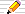icon means there is a Things to do investigation at the end of the section. The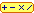icon means there is an interactive calculator in this section.

1·61803 39887 49894 84820 45868 34365 63811 77203 09179 80576 ..More..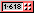# Powers of Phi

Here is part of the table of numerical properties of powers of Phi:

Here Phi = 1·6180339... = phi–1
and phi = 0·6180339... = Phi – 1 = 1/Phi = Phi–1
Phi
power
phi
power
A + B Phi C + D phireal
value
Phi5 phi-5 3 + 5 Phi 8 + 5 phi 11·0901699..
Phi4 phi-4 2 + 3 Phi 5 + 3 phi 6·8541019..
Phi3 phi-3 1 + 2 Phi 3 + 2 phi 4·2360679..
Phi2 phi-2 1 + 1 Phi 2 + 1 phi 2·6180339..
Phi1 phi-1 0 + 1 Phi 1 + 1 phi 1·6180339..
Phi0 phi0 1 + 0 Phi 1 + 0 phi 1·0000000..
Phi-1 phi1 -1 + 1 Phi 0 + 1 phi 0·6180339..
Phi-2 phi2 2 - 1 Phi 1 - 1 phi 0·3819660..
Phi-3 phi3 -3 + 2 Phi -1 + 2 phi 0·2360679..
Phi-4 phi4 5 - 3 Phi 2 - 3 phi 0·1458980..
Phi-5 phi5 -8 + 5 Phi -3 + 5 phi 0·0901699..
We can capture these relationships precisely in two formulae:

Phin = Fib(n–1) + Fib(n) Phi

Phin = Fib(n+1) + Fib(n) phi

It is not difficult to prove (by Induction) that these formulae are indeed correct. They both apply to negative n as well, if we extend the Fibonacci series backwards:
..., -8, 5, -3, 2, -1, 1, 0, 1, 1,2, 3, 5, 8, ...
where we still have the Fibonacci property: Fib(n) = Fib(n-1) + Fib(n-2) but it now holds for all values of n, positive, zero and negative!

Another property of this extended Fibonacci series of numbers is that

 Fib(–n) = –Fib(n) for even n Fib(–n) = Fib(n) for odd n

1·61803 39887 49894 84820 45868 34365 63811 77203 09179 80576 ..More..## Integers as sums of powers of Phi

In the table of powers of phi above, you will have noticed that the same multiples of Phi occur, sometimes positive and sometimes negative. For example, 2 phi occurs in both Phi3 = 3 + 2 phi and Phi-3 = -1 + 2 phi. If we subtract these two powers, the multiples of phi will disappear and leave us with an integer.
Similarly, 3 phi occurs in both Phi4 = 5 + 3 phi and Phi-4 = 2 - 3 phi. If we add these two powers, again the multiples of phi will cancel out and leave an integer.

Here are some more examples:

Phi1 + Phi–2 = (1 + 1 phi) + (1 – 1 phi) = 2
Phi2 + Phi–2 = (2 + 1 phi) + (1 – 1 phi) = 3
Phi4 + Phi–4 = (5 + 3 phi) + (2 – 3 phi) = 7
So we have expressed the integers 2, 3 and 7 as a sum of powers of Phi.

Because Phi0 is just 1, we can add 1 (=Phi0) to those numbers above and so represent 3 (again), 4 and 8 as a sum of powers of Phi.

We can also add combinations of these numbers and get other ones too. In all of them, we are writing the integer as a sum of different powers of Phi.

4 = 3 + 1 = (Phi2 + Phi–2) + Phi0
8 = 7 + 1 = (Phi4 + Phi–4) + Phi0
9 = 2 + 7 = (Phi1 + Phi–2) + (Phi4 + Phi–4)
10 = 3 + 7 = (Phi2 + Phi–2) + (Phi4 + Phi–4)
This reminds us of expressing numbers as :
• sums of powers of 2 (binary), or
• sums of powers of 3 (ternary), or
• sums of powers of 8 (octal) and, of course, the usual way using
• sums of powers of 10 (decimal)!

All the above are powers of an integer (2, 3, 8 or 10) but the really unusual thing here is that we are taking powers of Phi, an irrational number and adding them to get a pure integer!

A natural question now is:

Are all integers representable as sums of powers of Phi?
The answer is Yes! The number n is just n copies of Phi0 added together!!!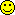So let's rephrase the question...
What we really meant to ask was how to do this using only powers of Phi and not repeating any power more than once in the sum (which is what we did in the examples above).

####Things to do1. 1 = Phi0 and
1 = Phi-1+ Phi-2 and
By expanding Phi-n (= phin) as Phi-(n+1)+Phi-(n+2) how many more ways can you find to sum powers pf Phi to a total of 1 if no power of Phi can be used more than once? e.g.
Phi-2 = Phi-3+Phi-4 so
1 = Phi-1+ Phi-2 expands to
1 = Phi-1+ Phi-3 + Phi-4
2. Try to express each of the following numbers as a sum of different powers of Phi each power occurring no more than once.
(a) on your calculator to see if you are approximately right but a more precise method is...
(b) to use the exact values by translating all the powers of Phi into sums of integers and multiples of Phi using the formula
Phin = Fib(n+1) + Fib(n) phi
so that you can check that all the multiples cancel out:
1. 5 as the sum of 2 and 3
2. 5 as the sum of 4 and 1
(use your answers to the first question using different representations of 1)
3. 6
4. 6 again, but find a different answer this time
5. 9 Find THREE different answers!
6. 10
7. 11
8. 12
9. each of the numbers from 13 to 20
3. Of your representations of number 6 in the previous question, which answer has the fewest powers of Phi?
4. Find a table of answers for all the values from 1 to 20 but all your answers should have the fewest number of powers in them.
From your answers to the above questions, it may look like many numbers can be expressed in Base Phi. Do you think that ALL whole numbers can be?
If you do, how would you try to convince someone of this?
If you do not, which integer do you think does NOT have a Base Phi representation? (Are you sure?)

1·61803 39887 49894 84820 45868 34365 63811 77203 09179 80576 ..More..# Base Phi Representations

Let's use what we learned on the Fibonacci Bases Page to write down our sums-of-distinct-powers-of-Phi representations of a number. As in decimal notation, the columns represent the powers of the Base, but for us the base is Phi, not 10. We have negative powers of Phi as well as positive ones, so, just as in decimal fractions, we need a "point" to separate the positive powers of Phi from the negative ones.

So if 1·25 in decimal means

 powers of 10: ... 3 2 1 0 . -1 -2 ... 1 . 2 5
= 1+ 2x10-1 + 5x10-2
then
2 = Phi1 + Phi-2 so 2 in Base Phi is
 powers of Phi: ... 3 2 1 0 . -1 -2 ... 1 0 . 0 1
= Phi1 + Phi–2
which we write as 2=10·01Phi to indicate that it is a Base Phi representation.

## There are Base Phi Representations for all whole numbers

You might like to convince yourself that, by successively adding 1's, if necessary applying the Expanding 1's process, then we can always find a way of representing ANY integer as sum of distinct powers of Phi. By applying the Reducing 1's process as often as necessary, we can then always find a base Phi representation that has the minimum number of 1's and no two of them will be next to each other.

Using the digits 0 and 1 only, we can express every integer as a sum of some powers of Phi

####Things to do1. How unusual is this property? Could we express every integer as sum of powers of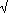2? (Hint: think about even powers of2)
2. What about powers of e=2.71828182... or=3.1415926535... or some other irrational value like Phi that has no integer power equal to an integer?

## Reducing the number of 1's in a Base Phi Representation

We haven't used much of the theory about Fibonacci numbers yet (those formulae further up this page). There are some interesting and relevant facts in the Formula for powers of Phi that we saw on the Phi's Fascinating Figures page. One of these was
Phin = Phin-1 + Phin-2
This tells us that, if ever we find two consecutive 1's in a Base Phi representation, we can replace them by an additional one in the column to the left

For instance,

3 = 2 + 1 = 10·01Phi + 1·0Phi = 11·01Phi
but we can replace the two consecutive 1's by a 1 in the phi2 column:
3 = 100·01Phi
Let's call this the Reducing 1's Process.

But what happens if we have three or more 1s next to each other?
Find the leftmost 11 and start there as there will always be two consecutive ones that have a zero on their left.
This will replace the two ones by zeros and so any following 11 will not have a zero in front of them.
We can always start with the leftmost pair of ones and then repeat the Reducing 1's Process on the new form if necessary until we eliminate all "11" from our representation.

Repeatedly applying the Reducing 1's process means that we can reduce a Base Phi representation until eventually we have no pairs of consecutive 1's

The base Phi representation of N with the least number of 1s is called the minimal representation of N
It follows from this definition that
1. Every minimal base Phi representation has no consecutive 1s
2. The minimal representation has the least number of 1s
3. Every minimal representation has the greatest number of 0s ignoring any leading and trailing 0s
4. Every whole number has a minimal base Phi representation

## Expanding the number of 1's in a Base Phi Representation

What if we get more than one of a certain power of Phi?
The solution here is to use the same formula but backwards, that is, replacing a 1 by 1's in the two columns to the right. So that, whenever we have
```       ...100... we can replace it by ...011...
```
Let's call this the Expanding 1's Process.
 EG 2 = 1+1 = 1·0Phi+1·0Phi Expanding the second 1·0 into 0·11: = 1·0Phi+0·11Phi Now we can add without getting more than 1 in any column: = 1·11Phi and we are ready to apply the Reducing 1's process: =10·01Phi
But every representation will end with a "1", which we can always expand into "011".
2 in base Phi is 10.01 and also 10.0011,
but we can expand the final 1 of 10.0011 to get the new form of 10.001011
and repeat on the final one again to give 10.00101011
and then 10.0010101011 and so on for ever!
All representations can be expanded to get an infinitely long tail of 010101...01011 !
To avoid this, we decide that
We will ignore base Phi representations that end ...011

But there is another case to consider too:
Any representation ending in ...101 is equivalent to ...10011 and now we can convert the initial 100 into 011 to get ...01111.
Note that the original and the final forms here have the same number of 0s.
For instance:

4 = 101.01 = 100.1111
10 = 1111.0101 = 1111.001111 = 1110.111111
This leads us to a decision to make about the base Phi representation for n which contains the most 1s: which we want to call the maximal base Phi representation.
For instance, 27 has the following representations with no consecutive 0s:-
27 = 111011.110101 is the shortest base Phi representation of 27.
Now we use the two expansions just explained to convert an ending of 101 into 01111:
27 = 111011.11001111 but now another "100" appears which we can convert to reduce the number of 0s:
27 = 111011.10111111 and this now has the least number of 0s in any base Phi representation of 27.

So here we define the maximal representation as follows

The base Phi representation of N that does not end in 011 and with the greatest number of 1s is called the maximal base Phi representation of N.
Several things follow from this definition:
1. Every maximal representation has no two consecutive 0s
2. Every maximal representation has the greatest number of 1s
3. Every maximal representation has the least number of 0s
4. Every whole number has a maximal base Phi representation
Note that if we had use "the least number of 0s" in the definition and not "the greatest number of 1s" then we would not have got a unique maximal representation:
for instance, 7 has representations 1010.1101 and 10101.01111 both of which contain the least number of 0s (three) but only the latter has the greatest number of 1s (seven) amongst all the base Phi reps of 7.

## Comparing the Minimal amd Maximal Representations

Here is a table of the minimal and maximal base Phi representations of 1 up to 30:
NMinimal rep
no 11s
fewest 1s
Maximal rep
no 00s
most 1s
11.1.
210.011.11
3100.0110.1111
4101.0111.1111
51000.1001101.1111
61010.0001111.0111
710000.00011010.101111
810001.00011011.101111
910010.01011101.101111
1010100.01011110.111111
1110101.01011111.111111
12100000.10100110101.111111
13100010.00100110111.011111
14100100.00100111010.110111
15100101.00100111011.110111
16101000.10000111101.110111
17101010.00000111111.010111
181000000.000001101010.10101111
191000001.000001101011.10101111
201000010.010001101101.10101111
211000100.010001101110.11101111
221000101.010001101111.11101111
231001000.100101110101.11101111
241001010.000101110111.01101111
251010000.000101111010.10111111
261010001.000101111011.10111111
271010010.010101111101.10111111
281010100.010101111110.11111111
291010101.010101111111.11111111
3010000000.101010011010101.11111111

 1 1. 2 10.01 1.11 3 100.01 4 11.1111 7 10000.0001 11 1111.111111 18 1000000.000001 29 111111.11111111
Some patterns are visible in the table above and shown on the left here:
2,1,3,4,7,11,18,29 are formed in the same way as the Fibonacci numbers, by adding the latest two to get the next, but instead of starting with 0 and 1 as we do for the Fibonacci Numbers, we start with 2 and 1. They are called the Lucas numbers, denoted L(n), and almost always appear where the Fibonacci numbers do!
Sum the two Fibonacci numbers on either side of each Fibonacci number and you generate each Lucas number:

L(n) = F(n–1) + F(n+1)
The formula for the Lucas numbers involves Phi and phi too:
L(n) = Phin + (–phi)n = Phin + (–Phi)–n = Phin + (–1)n Phi–n For instance
L(2) = 3 = Phi2 + Phi–2 and so is 100.01 in Base Phi.
L(4) = 7 = Phi4 + Phi–4 and so is 10000.0001 in Base Phi.
But for L(0) = 2, we get Phi0 + Phi-0 so the two powers of Phi are the same and 2 is 2 Phi0.
The significance of the maximal representation is seen in the following table of sums all with a total of eleven:
 1+ 1 2+ 10.01 3+ 100.01 4+ 101.01 10 1110.11 9 1101.101111 8 1011.101111 7 1010.1 11 1111.11 11 1111.111111 11 1111.111111 11 1111.11
Since 11, the fifth Lucas number, L(5), has a maximal representation of 1111.111111 with no zeros then the 1s of the top number in the sum are the 0s in the bottom number.
Sometimes we need to change the final 1 (of either number) into 011 to make the number of phigits after the (phigital-)point the same as in 5+6=11 :
 5+ 1000.1001  = 1000.100011+ 6 111.0111 111.0111 11 1111.111111
The next number with a maximal representation with no zeros is L(7), 29, and you will notice the same patterns between 1 and 28, 2 and 27, 3 and 26, etc where the "h0les" in the larger numbers are "f1lled" in the smaller and vice-versa.

1·61803 39887 49894 84820 45868 34365 63811 77203 09179 80576 ..More..## All forms of Base Phi representation

By converting "100" to "011" (or vice-versa) in any Base Phi representation, we get another valid base Phi form.
As we saw above when converting to the maximal form (no consecutive 0s) we can always convert the final "1" to "011" and so continue the expansion of N in Base Phi for ever. So we will only count finite Base Phi forms which do not end in "011" to prevent this.
Here is a table of equivalent Base Phi forms for the values 1 to 11:
1234567891011
1 10.01
1.11
100.01
11.01
10.1111
101.01
100.1111
11.1111
1000.1001
1000.0111
110.1001
110.0111
101.1111
1010.0001
1001.0111
1001.1001
111.1001
111.0111
10000.0001
1100.0001
1011.0001
1010.1101
1010.101111
10001.0001
10000.1101
10000.101111
1101.0001
1100.1101
1100.101111
1011.1101
1011.101111
10010.0101
10010.001111
10001.1101
10001.101111
1110.0101
1110.001111
1101.1101
1101.101111
10100.0101
10100.001111
10011.0101
10011.001111
10010.111111
1111.0101
1111.001111
1110.111111
10101.0101
10101.001111
10100.111111
10011.111111
1111.111111
The counts here are 1, 2, 3, 3, 5, 5, 5, 8, 8, 8, 5 all of which should look familiar to you by now! However the pattern does not continue and is not even solely Fibonacci numbers.

## A Phigits Calculator

C A L C U L A T O R
 Convert up to to Base Phi Convert 100000.011111

R E S U L T S

1·61803 39887 49894 84820 45868 34365 63811 77203 09179 80576 ..More..# Other names for Base Phi

Representations of an integer n as a sum of different powers of Phi are aclled the Base Phi representation of n on this page.
Other names that have been suggested are
• Phigital: compare with digital for Base Ten;
• Phinary: compare with Binary since we are also using just the digits 0 and 1 but to base Phi [with thanks to Marijke van Gans for this term];
• expressing a number in Phigits [With thanks to Prof Jose Glez-Regueral of Madrid for mentioning this one.]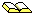Some of the above originally appeared in an article by George Bergman, in the Mathematics Magazine 1957, Vol 31, pages 98-110, where he also gives pencil-and-paper methods of doing arithmetic in Base Phi.C. Rousseau The Phi Number System Revisited in Mathematics Magazine 1995, Vol 68, pages 283-284.© 1996-2015 Dr Ron Knott